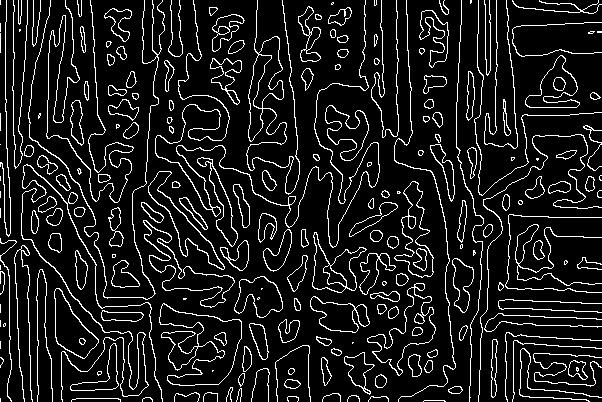Sobel and Laplacian of Gaussian(LoG) Edge Detectors

These programs perform edge detection on an input image. The Sobel edge detector uses 2 simple 3x3 filters to detect edges in the x and y directions. These outputs are combined into an output image and thresholded at various values to keep more or less of the edges. The LoG edge detector calculates a discrete filter from continuous equations given an input sigma which determines the width. This filter is then applied to the image and pixels are set in the output image corresponding to zero-crossings in the filtered image. This gives the continuous contour-like edges seen below.
Original ImageSobel (threshold 10)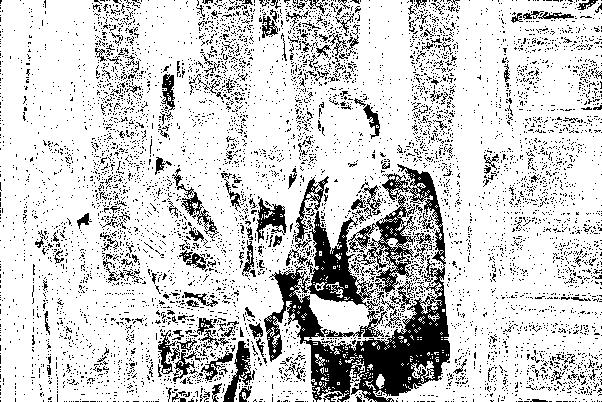Sobel (threshold 100)Sobel (threshold 255)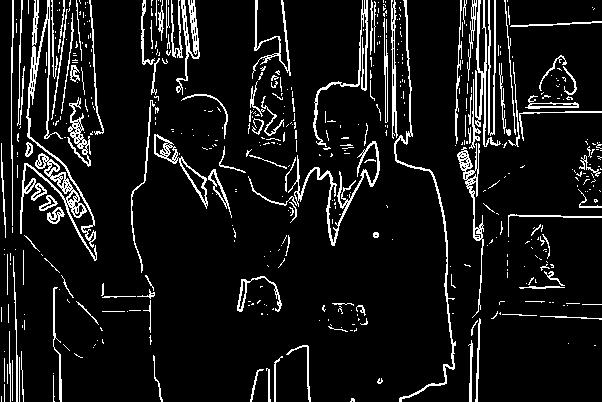Laplacian of Gaussian (sigma=1.0)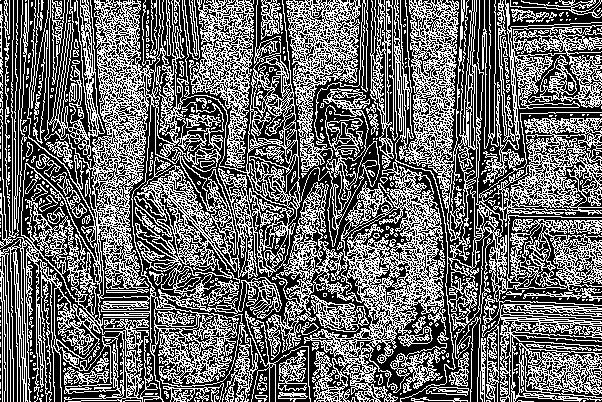Laplacian of Gaussian (sigma=2.0)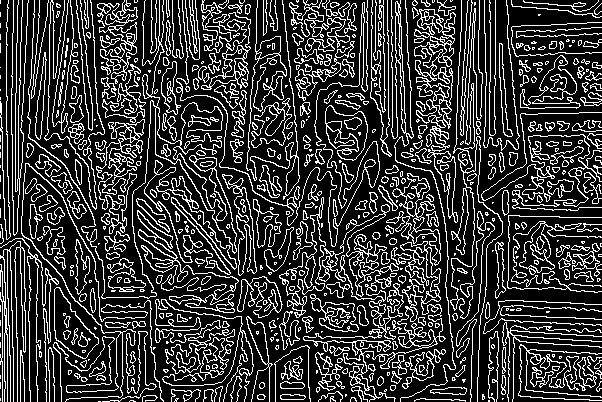Laplacian of Gaussian (sigma=4.0)